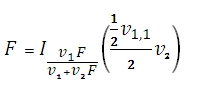# SQL Server F.DIST function

Updated: 9 August 2010

Use FDIST to calculate the F probability distribution. A frequent use of the F-distribution is to test whether two observed samples have the same variance. FDIST calculates the cumulative distribution function. The F-distribution cdf is given in terms of the incomplete beta function:Syntax
SELECT [wctStatistics].[wct].[FDIST] (
<@X, float,>
,<@Degrees_freedom1, float,>
,<@Degrees_freedom2, float,>)
Arguments
@X
is the value to be evaluated by the function. @X is an expression of type float or of a type that can be implicitly converted to float
@Degrees_freedom1
is the numerator degrees of freedom. @Degrees_freedom1 is an expression of type float or of a type that can be implicitly converted to float
@Degrees_freedom2
is the denominator degrees of freedom. @Degrees_freedom2 is an expression of type float or of a type that can be implicitly converted to float
Return Types
float
Remarks
·         If @X < 0, FDIST returns an error.
·         @Degrees_freedom1 and @Degrees_freedom2  are truncated to zero decimal places.
·         If @Degrees_freedom1 < 1 or @Degrees_freedom1 > 10^10, FDIST returns an error.
·         If @Degrees_freedom2 < 1 or @Degrees_freedom2 > 10^10, FDIST returns an error.
·         FDIST = BETADIST(@Degrees_freedom2 /(@Degrees_freedom2 +@Degrees_freedom1 *@X), @Degrees_freedom2 /2, @Degrees_freedom1 /2)
Examples

select
wct.FDIST(3.217174547, 6 ,10)

This produces the following result
----------------------
0.0500000000172287

(1 row(s) affected)

select

This produces the following result
----------------------
0.0500000000172289

(1 row(s) affected)

### SupportCopyright 2008-2023 Westclintech LLC         Privacy Policy        Terms of Service